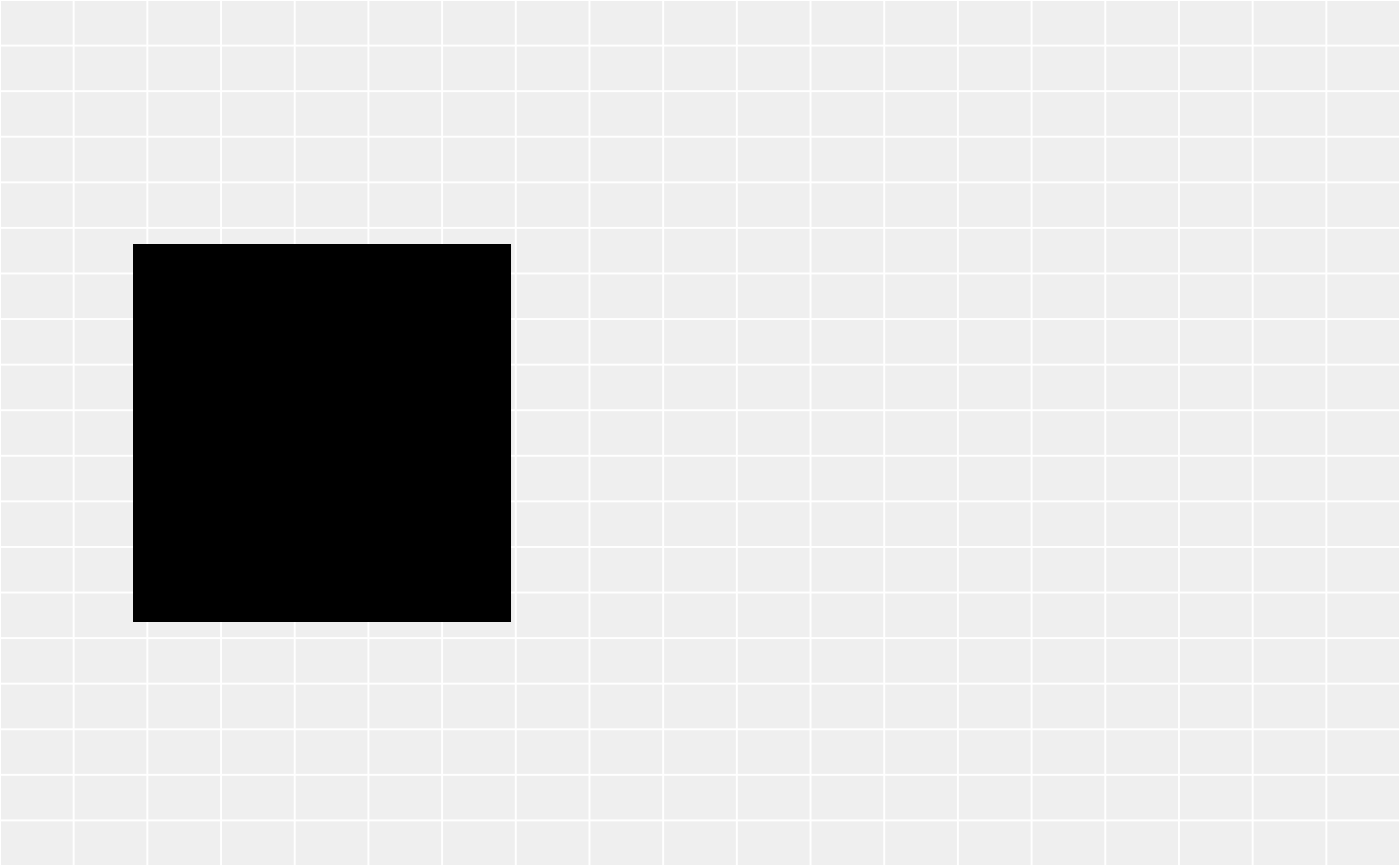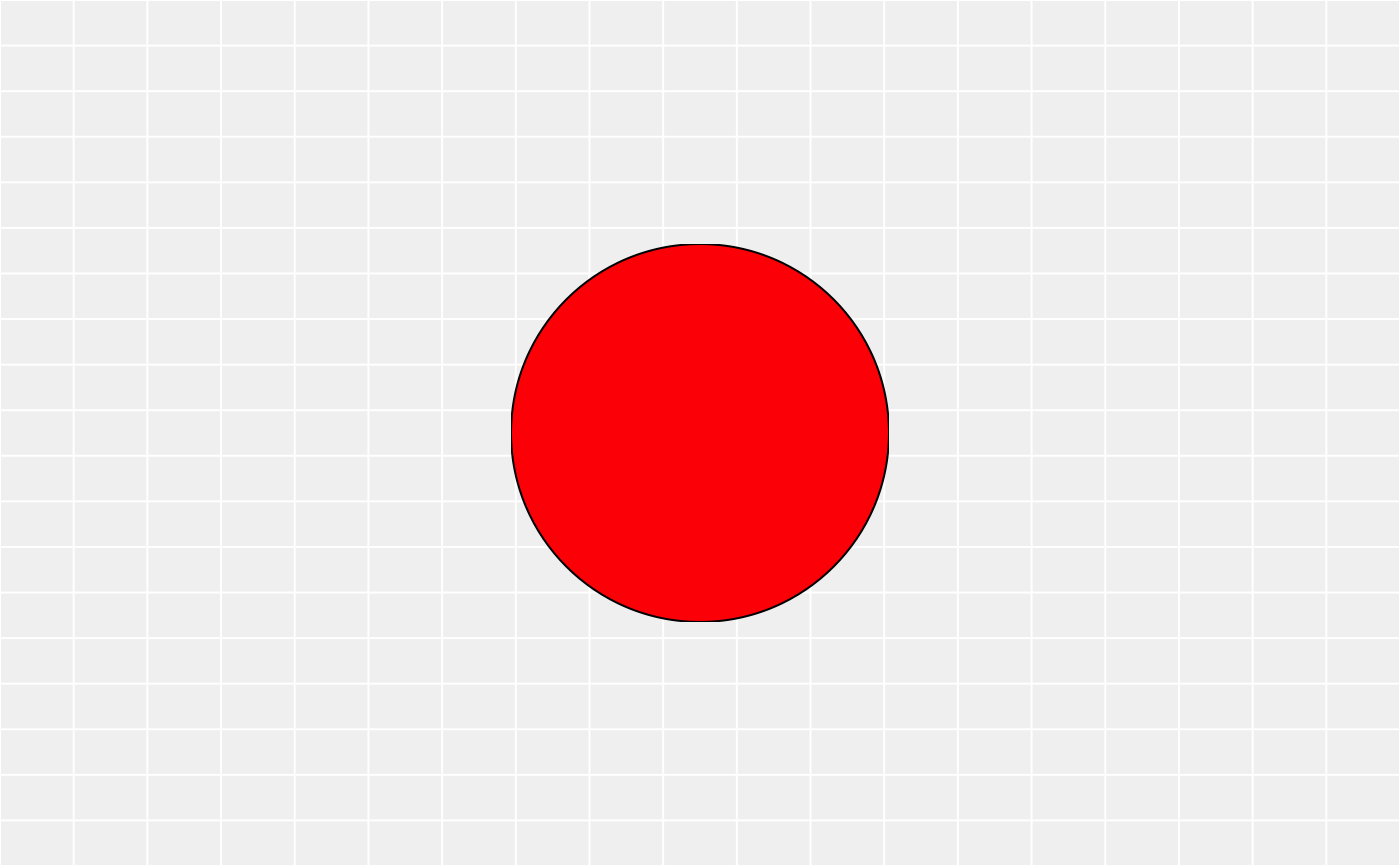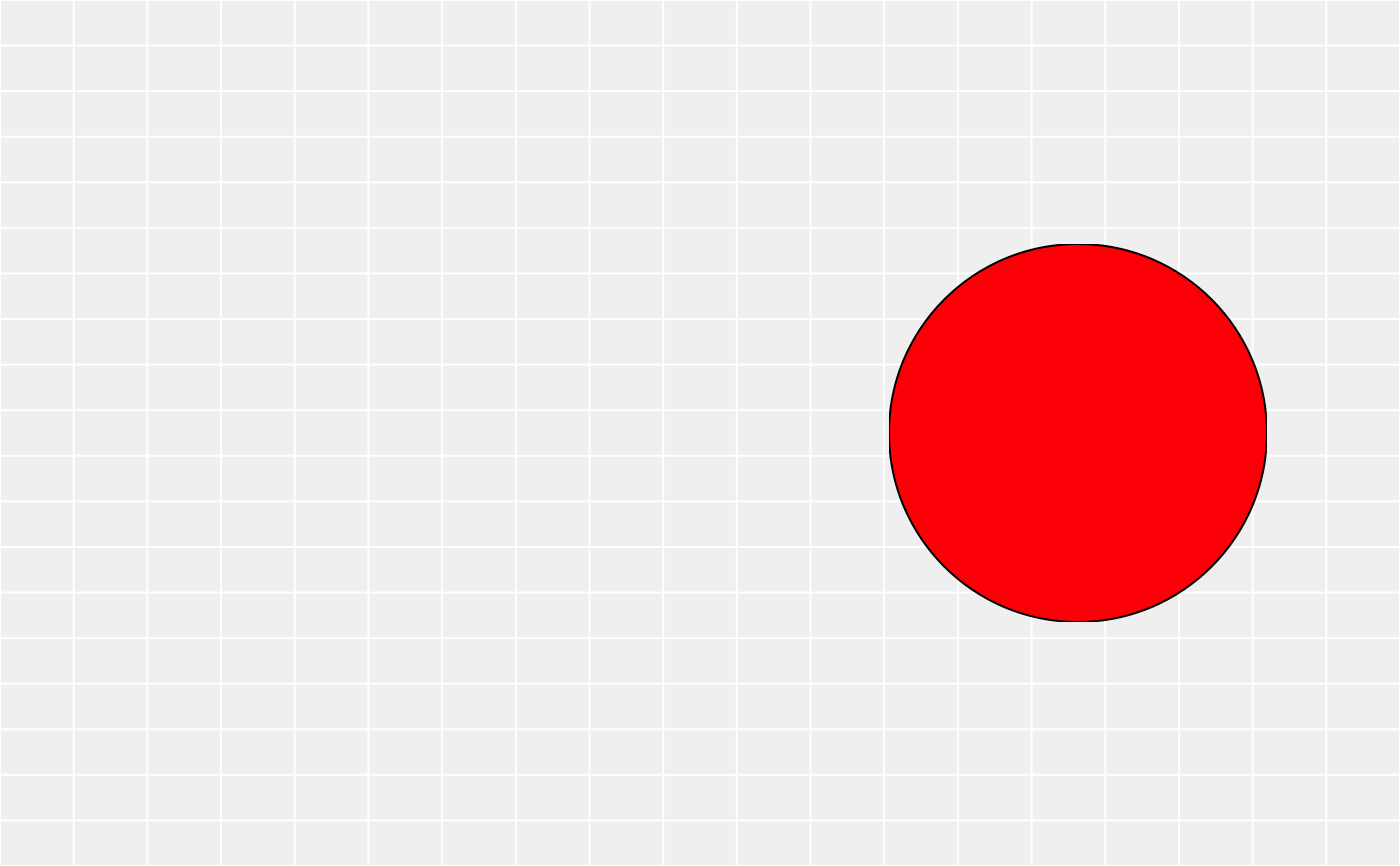Filter cells by name.

gtable_filter(x, pattern, fixed = FALSE, trim = TRUE)

## Arguments

x a gtable object character string containing a regular expression (or character string for fixed = TRUE) to be matched in the given character vector. Coerced by as.character to a character string if possible. If a character vector of length 2 or more is supplied, the first element is used with a warning. Missing values are allowed except for regexpr and gregexpr. logical. If TRUE, pattern is a string to be matched as is. Overrides all conflicting arguments. if TRUE, gtable_trim() will be used to trim off any empty cells.

## Examples

library(grid)
gt <- gtable(unit(rep(5, 3), c("cm")), unit(5, "cm"))
rect <- rectGrob(gp = gpar(fill = "black"))
circ <- circleGrob(gp = gpar(fill = "red"))

gt <- gtable_add_grob(gt, rect, 1, 1, name = "rect")
gt <- gtable_add_grob(gt, circ, 1, 3, name = "circ")

plot(gtable_filter(gt, "rect"))plot(gtable_filter(gt, "rect", trim = FALSE))plot(gtable_filter(gt, "circ"))plot(gtable_filter(gt, "circ", trim = FALSE))